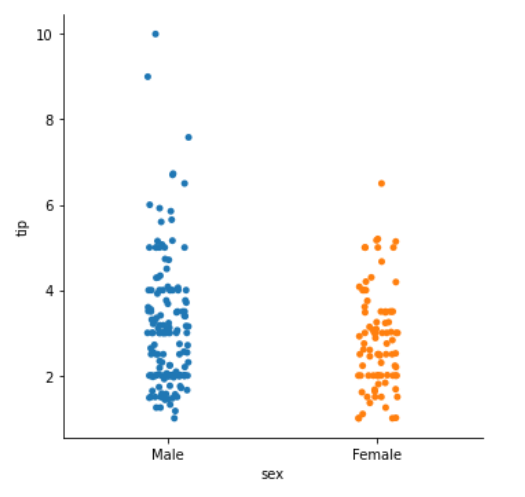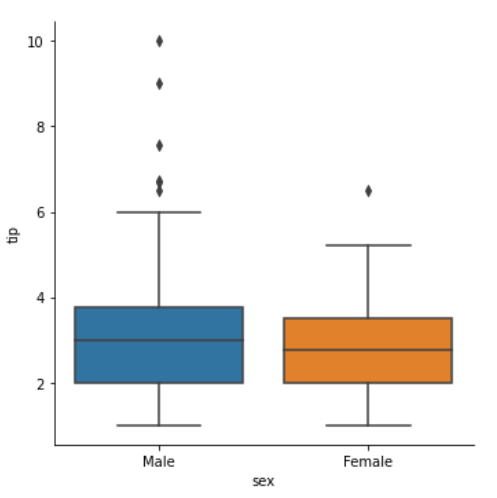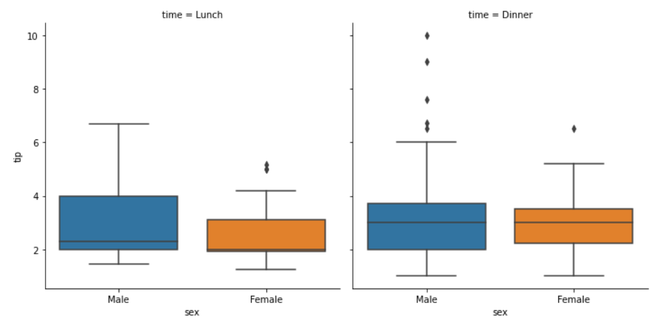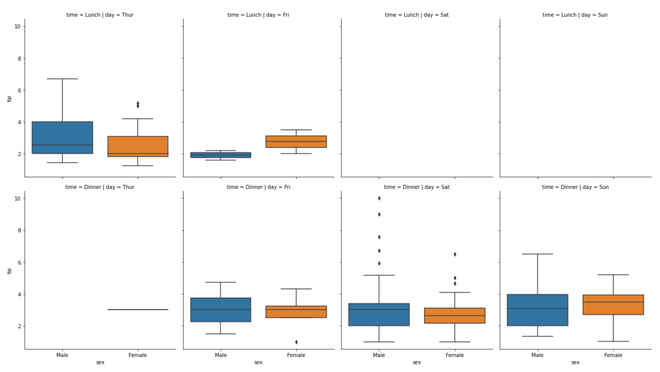Open in App
Not now

# How To Make Simple Facet Plots with Seaborn Catplot in Python?

• Last Updated : 26 Nov, 2020

Seaborn is an amazing visualization library for statistical graphics plotting in Python. It provides beautiful default styles and color palettes to make statistical plots more attractive. It is built on the top of matplotlib library and also closely integrated into the data structures from pandas.
Seaborn aims to make visualization of the central part of exploring and understanding data. It provides dataset-oriented APIs, so that we can switch between different visual representations for the same variables for a better understanding of the dataset.

Facet plots, where one subsets the data based on a categorical variable and makes a series of similar plots with the same scale.

We can make facet plots in Python in multiple ways. In this post, we will see an example of making simple facet plots using Seaborn’s Catplot() method. It is mostly used to visualize when there is a numerical variable and a corresponding categorical variable.

### Steps Required

1. Import Libraries.
2. Import or create data.
3. Use Catplot() method with the facet plot.
4. Use other arguments for better visualization.

### Dataset Used

The dataset used in the below example is https://www.kaggle.com/ranjeetjain3/seaborn-tips-dataset

Example 1:

## Python3

 `# importing packages ` `import` `seaborn ` ` `  `# load data ` `tip ``=` `seaborn.load_dataset(``'tips'``) ` ` `  `# create catplot facetplot object ` `seaborn_facetgrid_object ``=` `seaborn.catplot( ` `    ``x``=``'sex'``, ` `    ``y``=``'tip'``, ` `    ``data``=``tip ` `) ` `# show plot ` `seaborn_facetgrid_object `

Output:Example 2:

## Python3

 `# importing packages ` `import` `seaborn ` ` `  `# load data ` `tip ``=` `seaborn.load_dataset(``'tips'``) ` ` `  `# create catplot facetplot object ` `seaborn_facetgrid_object ``=` `seaborn.catplot( ` `    ``x``=``'sex'``, ` `    ``y``=``'tip'``, ` `    ``kind``=``'box'``, ` `    ``data``=``tip ` `) ` `# show plot ` `seaborn_facetgrid_object `

Output:Example 3:

## Python3

 `# importing packages ` `import` `seaborn ` ` `  `# load data ` `tip ``=` `seaborn.load_dataset(``'tips'``) ` ` `  `# create catplot facetplot object ` `seaborn_facetgrid_object ``=` `seaborn.catplot( ` `    ``x``=``'sex'``, ` `    ``y``=``'tip'``, ` `    ``col``=``'time'``, ` `    ``kind``=``'box'``, ` `    ``data``=``tip ` `) ` `# show plot ` `seaborn_facetgrid_object `

Output:Example 4:

## Python3

 `# importing packages ` `import` `seaborn ` ` `  `# load data ` `tip ``=` `seaborn.load_dataset(``'tips'``) ` ` `  `# create catplot facetplot object ` `seaborn_facetgrid_object ``=` `seaborn.catplot( ` `    ``x``=``'sex'``, ` `    ``y``=``'tip'``, ` `    ``row``=``'time'``, ` `    ``col``=``'day'``, ` `    ``aspect``=``0.9``, ` `    ``dodge``=``False``, ` `    ``kind``=``'box'``, ` `    ``data``=``tip ` `) ` `# show plot ` `seaborn_facetgrid_object `

Output:My Personal Notes arrow_drop_up
Related Articles